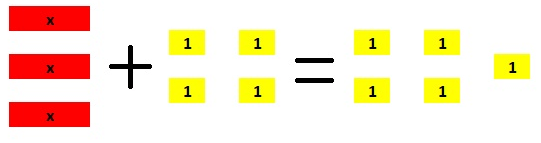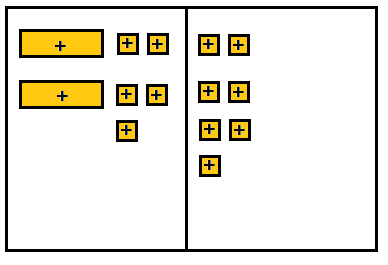# Texas Go Math Grade 7 Lesson 8.1 Answer Key Writing Two-Step Equations

Refer to our Texas Go Math Grade 7 Answer Key Pdf to score good marks in the exams. Test yourself by practicing the problems from Texas Go Math Grade 7 Lesson 8.1 Answer Key Writing Two-Step Equations.

## Texas Go Math Grade 7 Lesson 8.1 Answer Key Writing Two-Step Equations

Modeling Two-Step Equations

You can use algebra tiles to model two-step equations.Use algebra tiles to model 3x – 4 = 5.
A. How can you model the left side of the equation?
B. How can you model the right side of the equation?
C. Use algebra tiles or draw them to model the equation on the mat.Reflect

Question 1.
What If? How would you change the equation in the Explore Activity to model -3x + 4 = 5?
In order to change the model for the given equation and model the equation -3x + 4 = 5 use the following:
• • Instead of using 3 yellow tiles for the positive variable, use 3 red tiles for the negative variabLe to show – 3x
• • Instead of using 4 red tiles for -1, use 4 yelLow tiles for 1.
The model for the equation 3x + 4 = 5 is:Change the yellow tiles for the positive variable to red tiles for negative variable.
Change red tiles for -1 to yellow tiles for 1.

Question 2.
Multiple Representations Why would this equation for finding the monthly fee be difficult to model with algebra tiles?
It will be difficult because we would need so many tiles.

Question 3.
Can you rewrite the equation in the form 52m = 460? Explain.
No, we can not. On the left side of the equation we have number and variable m, and we can not sum the number and variable m.

Question 4.
Billy has a gift card with a $150 balance. He buys several video games that cost$35 each. After the purchases, his gift card balance is $45. Write an equation to help find out how many video games Billy bought. Answer: Let x be the number of video games Billy bought.$150 – $35 ∙ x =$45

Question 5.
Write a real-world problem that can be represented by 10x + 40 = 100.
Bart go to store to buy a tennis balls and a racket. He buys one racket that cost $10 and$10 tennis balls. The bill was $100. how much does one tennis ball cost? Texas Go Math Grade 7 Lesson 8.1 Guided Practice Answer Key Draw algebra tiles to model the given two-step equation. (Explore Activity) Question 1. 2x + 5 = 7Answer:On the left side of the mat we put 2 positive variable and 5 + 1 tiles. On the right side we put 7 + 1 tiles. Question 2. -3 = 5 – 4xAnswer:On the left side of the mat we put 3 – 1 tiles. On the right side we put 4 negative variable and 5 + 1 tiles. Question 3. A group of adults plus one child attend a movie at Cineplex 15. Tickets cost$9 for adults and $6 for children. The total cost for the movie is$78. Write an equation to find the number of adults in the group. (Example 1)
Let x be the number of adults.
$9 ∙ x –$6 = $78 Question 4. Break down the equation 2x + 10 = 16 to analyze each part. (Example 2) x is ________ of the problem. 2x is the quantity you are looking for _________. + 10 means 10 is ________. = 16 means the __________ is 16. Answer: – Solution. – Is multiplied by 2. – Added to 2x. – The result Question 5. Write a corresponding real-world problem to represent 2x – 125 = 400. (Example 2) Answer: John bought two bicycles and get discount of$124. Total cost after discount is $100. How much is the cost of one bicycle? Essential Question Check-In Question 6. Describe the steps you would follow to write a two-step equation you can use to solve a real-world problem. Answer: The steps in writing a two-step equation to solve a real-worLd problem: 1. Read the problem carefully and identify what is needed. Assign a variable for the said needed information. 2. Write down the important information that can be used to help to write an equation. 3. Use words ¡n the problem to tie the information together. Translate these words into numbers and variables in order to make an equation. Texas Go Math Grade 7 Lesson 8.1 Independent Practice Answer Key Question 7. Describe how to model -3x + 7 = 28 with algebra tiles. Answer: On the left side we put 3 negative variable and 7 + 1 tiles. On the right side we put 28 + 1 tiles. To solve the equation, remove 7 + 1 tiles from each side. Then, divide each side into 3 equal groups. The solution will be x = -7. Question 8. Val rented a bicycle while she was on vacation. She paid a flat rental fee of$55.00, plus $8.50 each day. The total cost was$123. Write an equation you can use to find the number of days she rented the bicycle.
At first, Val paid $55. and then plus$8.5 per day for a bicycle. Let x be the number of days Val rented the bicycle.
$55 +$8.5 ∙ x = $123 Question 9. A restaurant sells a coffee refill mug for$6.75. Each refill costs $1.25. Last month Keith spent$31.75 on a mug and refills. Write an equation you can use to find the number of refills that Keith bought.
Coffee refill mug costs $6.75, and each refill costs$1.25. Let x be the number of refills that Keith bought.
$6.75 +$1.25 ∙ x = $31.75 Question 10. A gym holds one 60-minute exercise class on Saturdays and several 45-minute classes during the week. Last week all of the classes lasted a total of 285 minutes. Write an equation you can use to find the number of weekday classes. Answer: During the week exercise class Lasts 4 – minutes, and on Saturday 60 – minutes. Let x be the number of weekday classes. 60 + 45 ∙ x = 285 Question 11. Multiple Representations There are 172 South American animals ¡n the Springdale Zoo. That is 45 more than half the number of African animals in the zoo. Write an equation you could use to find n, the number of African animals in the zoo. Answer: Springdale zoo has 45 more animals than half the number of African animals in the zoo. Let n be the number of African animales in the zoo. $$\frac{n}{2}$$ + 45 = 172 Question 12. A school bought$548 in basketball equipment and uniforms costing $29.50 each. The total cost was$2,023. Write an equation you can use to find the number of uniforms the school purchased.
Basketball equipment costs $548 and each uniform$29.5. Let x be the number of uniforms the school purchased.
$548 +$29.5 ∙ x = $2023 Question 13. Financial literacy Heather has$500 in her savings account. She withdraws $20 per week for gas. Write an equation Heather can use to see how many weeks it will take her to have a balance of$220.
Heather spends. per week, $20 for gas, from hers saving account. Let x be the number of weeks.$500 – $20 ∙ x =$220

Question 14.
Critique Reasoning For 9x + 25 = 88, Deena wrote the situation “I bought some shirts at the store for $9 each and received a$25 discount. My total bill was $88. How many shirts did I buy?” a. What mistake did Deena make? Answer: She received discount, hence. she paved$25 less, so she have to subtract $25, not to add. b. Rewrite the equation to match Deena’s situation. Answer: Deena bought some shirts that costs$9, and got the $25 discount. Let x be the number of shirts Deena bought. c. How could you rewrite the situation to make it fit the equation? Answer:$9 ∙ x – $25 =$88

Question 15.
Multistep Sandy charges each family that she babysits a flat fee of $10 for the night and an extra$5 per child. Kimmi charges $25 per night, no Work Area matter how many children a family has. a. Write a two-step equation that would compare what the two girls charge and find when their fees are the same. Answer: Let x be the number of children. Let’s write the equation. Left side of equation represents how much Sandy charges for babysitting per night, and the right side represents who much Kimmi charges per night.$10 + $5 ∙ x =$25
Subtract from both sides $10. -$10 + $10 +$5 ∙ x = -$10 +$25
$5 ∙ x =$15
Divide both sides by $5. $$\frac{x \cdot \ 5}{\ 5}=\frac{3 \cdot \ 5}{\ 5}$$ Their fees are the same when they babysit 3 children. b. How many children must a family have for Sandy and Kimmi to charge the same amount? Answer: 3 children c. The Sanderson family has five children. Which babysitter should they choose if they wish to save some money on babysitting, and why? Answer: They should choose Kimmi because she charges$25 per night. no matter how many children she babysits, and Sandy charges flat fee of $10 pills extra$5 per child, which would be
$10 + 5 ∙$5 = $35. H.O.T. Focus on Higher Order Thinking Question 16. Analyze Relationships Each student wrote a two-step equation. Peter wrote the equation 4x – 2 = 10, and Andres wrote the equation 16x – 8 = 40. The teacher looked at their equations and asked them to compare them. Describe one way in which the equations are similar. Answer: The equations are similar, because any equation can be multiplied or divided by any number and still be the same. In this case, if we multiply Peter’s equation by 4, we get the Andres’s equation. Hence, these two equation are equal. Question 17. What’s the Error? Damon has 5 dimes and some nickels in his pocket, worth a total of$1.20. To find the number of nickels Damon has, a student wrote the equation 5n + 50 = 1.20. Find the error in the student’s equation.